GeeksforGeeks App
Open AppBrowser
Continue

# What are Area Formulas for different Geometric Shapes?

There are many geometrical shapes/figures available and we can find the area for each figure using different formulas based on their shape. The area of a shape can be defined as a space enclosed by a closed geometric figure in a two-dimensional plane. In this article, we are finding the area of a few geometric shapes using different formulas.

### Area of Triangle

The area of a triangle is calculated by multiplying one half the base times the height.

Area of Triangle = (1/2) × Base × Height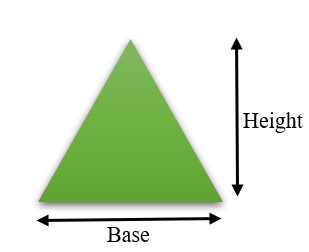Example: Find the area of a triangle with a height of 6cm and a base of 4cm.

Solution:

To find the area of triangle, we had a formula i.e., (1/2) × base × height

So, for this Triangle Base = 4 & Height = 6

Area = (1/2) × 4 × 6

= (1/2) × 24

= 12

So the area of the given triangle is 12cm2.

### Area of Square

In a square, the length of all sides are the same and to find the area for the square we need to find the square of the length of a side.

Area of Square = Side × Side = Side2

Example: Find the area of a square with each side of length 4cm.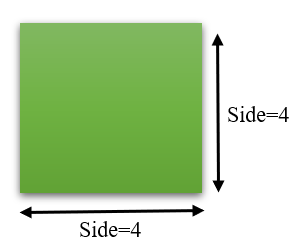Solution:

From given figure, We have square in which length of each side is 4cm. We can find area to it by

Area of Square = Side × Side

= 4 × 4

= 16

So for the given square the area is 16cm2.

### Area of Rectangle

The area of a rectangle can be calculated by multiplying its length and width of it. Because in rectangle opposite faces have the same length(measurements).

Area of Rectangle = length × width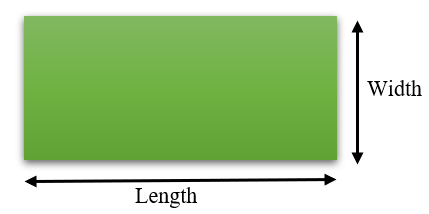Note: The formula for area of parallelogram is same as of formula for finding area of rectangle.

Example: What is the area of the rectangle of length 10 cm and width 5cm.

Solution:

From given figure,

length = 10cm

width = 5cm

Area of rectangle = length × width

= 10 × 5

= 50

So, the area of given rectangle is 50cm2

### Area of Circle

The area of a circle can be calculated by using the formula πr2 where r is the radius of a circle and π = 22/7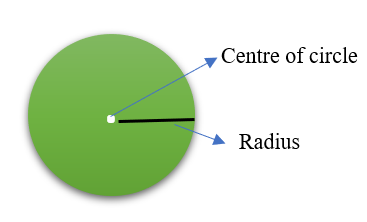Example: Find the circle area with a radius of 5cm.

Solution:

From given data,

area of circle = π × radius2

= (22/7) × 52

= (22/7) × 25

= 78.57 approximately

So the area of circle is 78.57 cm2

### Area of Trapezoid

In a trapezoid, we have 2 different lengths of bases i.e., top and bottom lengths. To find the area of a trapezoid the formula is half the sum of lengths of two bases times height.

Area of Trapezoid = (1/2) × (base1 + base2) × height

Example: Find the area of below given Trapezoid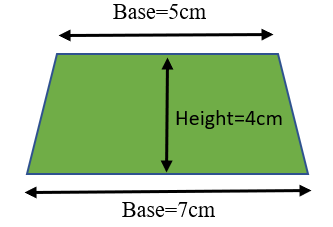Solution:

From the given figure,

length of Base1 = 7cm

length of Base2 = 5cm

height of trapezium = 4cm

Area of Trapezium = (1/2) × (base1 + base2) × height

= (1/2) × (5 + 7) × 4

= (1/2) × 12 × 4

= 24cm2

So area of given trapezium is 24cm2.

### Area of Eclipse

Before knowing the formula for finding the eclipse, we need to know about the eclipse.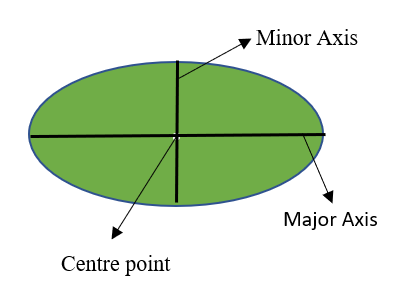In geometry, an eclipse is in the shape of an oval and it has a major axis and minor axis as shown in the figure. To find the area of the eclipse we need to perform multiplication among the length of the semi-major axis, semi-minor axis and π.

Area of eclipse = π × (Semi-major axis length) × (Semi-minor axis length)

Semi-major axis length = length of major axis/2

Semi-minor axis length = length of minor axis/2

Example: What is the area of the eclipse when its length of the major axis and the minor axis is 6cm and 3cm respectively.

Solution:

From the given data,

length of major axis = 6

length of minor axis = 3

length of semi major axis = length of major axis/2

= 6/2

= 3

length of semi minor axis = length of minor axis/2

= 3/2

=1.5

area of eclipse = π × (length of semi major axis) × (length of semi minor axis)

= (22/7) × 3 × 1.5

= 14.14 approximately

So for the given eclipse area is 14.14cm2

### Area of Rhombus

The area of the Rhombus is calculated by finding half of the product of the lengths of two diagonals of a rhombus. The formula is given by:

Area of Rhombus = (1/2) × Length(Diagonal1) × Length(Diagonal2)

Pictorial representation of rhombus is given below: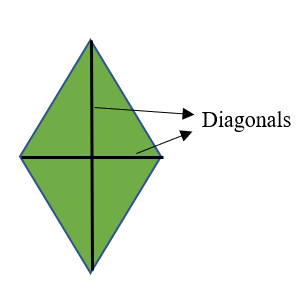Example: Find the area of the rhombus where the lengths of diagonals are 3cm and 6cm.

Solution:

From the given data,

Length of Diagonal1 = 3cm

Length of Diagonal2 = 6cm

Area of Rhombus = (1/2) × Length(Diagonal1) × Length(Diagonal2)

= (1/2) × 3 × 6

= 9

So the area of rhombus is 9cm2.

My Personal Notes arrow_drop_up
Related Tutorials